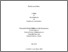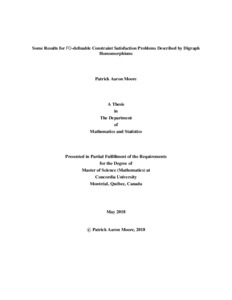Title:

# Some Results for FO-definable Constraint Satisfaction Problems Described by Digraph Homomorphisms

Moore, Patrick Aaron (2018) Some Results for FO-definable Constraint Satisfaction Problems Described by Digraph Homomorphisms. Masters thesis, Concordia University.Preview
Text (application/pdf)
Moore_MSc_F2018.pdf - Accepted Version
417kB

## Abstract

Constraint satisfaction problems, or CSPs, are a naturally occurring class of problems which involve assigning values to variables while respecting a set of constraints. When studying the computational and descriptive complexity of such problems it is convenient to use the equivalent formulation, introduced by Feder and Vardi, that CSPs are homomorphism problems. In this context we ask if there exists a homomorphism to some target structure. Using this view many tools and ideas have been introduced in combinatorics, logic and algebra for studying the complexity of CSPs. In this thesis we concentrate on combinatorics and give characterization results based on digraph properties. Where previous studies focused on CSPs defined by a single digraph with lists we extend our relational structures to consist of many binary relations which each individually describe a distinct digraph on the structures universe. A majority of our results are obtained by using an algorithm introduced by Larose, Loten and Tardif which determines whether a structure defines a CSP whose homomorphism problem can be represented by first order logic. Using this tool we begin by completely classifying which of these structures are FO-definable when each of the relations defines a transitive tournament. We then generalize a characterization theorem, first given by Lemaître, to include structures containing any finite number of digraph relations and lists. We conclude with examples of obstructions and properties that can determine if a particular relational structure has a CSP which is FO-definable and how to construct such structures.

Divisions: Concordia University > Faculty of Arts and Science > Mathematics and Statistics Thesis (Masters) Moore, Patrick Aaron Concordia University M. Sc. Mathematics 31 May 2018 Larose, Benoit 983926 PATRICK AARON MOORE 12 Nov 2018 18:06 12 Nov 2018 18:06
All items in Spectrum are protected by copyright, with all rights reserved. The use of items is governed by Spectrum's terms of access.

Repository Staff Only: item control pageResearch related to the current document (at the CORE website)
Back to top